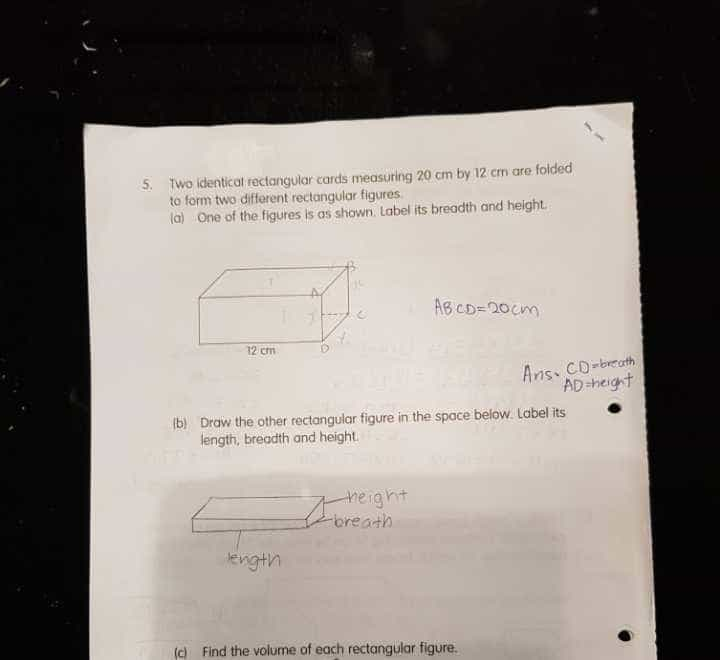# QuestionNeed help on Qn C) Find the volume of any cuboid created from a rectangular piece of paper size 20 x 12cm

Source: School

This is likely a workbook exercise.

20cm can be made of up (#1) 5+5+5+5 (cross section is a square), or (#2) 6+4+6+4, or (#3) 9+1+9+1 or (#4) 6.5+3.5+6.5+3.5, etc

Volume will be length 12cm x cross sectional area so equal to (#1) 12 x 5 x 5 = 300 sq cm, or (#2) 12 x 6 x 4 = 288 sq cm, or (#3) 12x9x1=108 sq cm, or (#4) 12×6.5×3.5=273 sq cm, etc.

This exercise is probably to let the kids play with folding same sheets of paper can fold into different rectagular figures which will have different volume each. There is no single answer to this question as long as the rectangle is labelled with correct dimensions and volume is correctly computed.